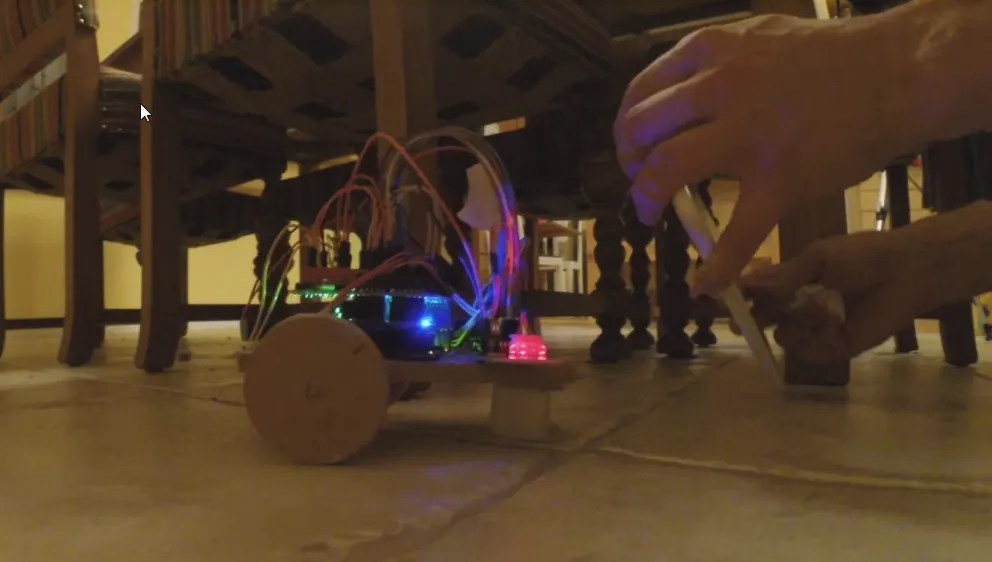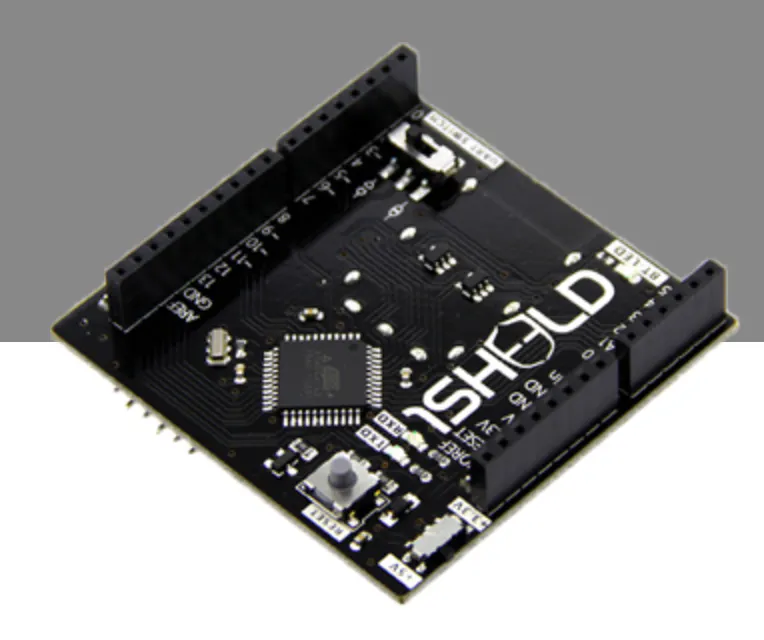Project tutorial# Bluetooth Steering wheel for a robot using 1Sheeld

A simple bumper robot with a simple steering wheel using a 1Sheeld, and an Android tablet/phone.

• 3,558 views
• 1 comment
• 13 respects

## Components and supplies

 28BYJ-48 Stepper motors, costs around 2\$ on ebay a piece, or buy many for less.
×2
 74HC595 Shift register
×11Sheeld
×1
 Micro switch Some kind of button, these are the simplest ones, buy 100pc for about a 1\$ on ebay.com
×1Arduino UNO Can be bought a cheap China copy for around 4-6\$
×1

## Necessary tools and machines

 Glue gun

For demonstration of all the functionality see the last 8 minutes of the last video with the 1Sheeld. :) Lots of fun with both me and the kids driving and bumping.

So I made a robot. It is a really simple robot, it is a bumper robot. For anyone who wants to get into robotics with an Arduino, I could really recommend this project as it is cheap (less than 10\$ total (not including the 1Sheeld (not needed for bumper robot functionality))) and very easy, with easy to understand code. An instructive video for how to make the Platform build and the initial code is below:

How to build the Arduino Bumper Robot.

Next, I thought I needed to use a bit fewer pins (above build uses 8! pins for the motors). So by adding a 74HC595 I managed to get the port count down from previous 8 pins to three pins. Here is another video showing this step (This video also gives you great insight in how Binary counting works):

And finally I wanted to add a little more interaction with the robot, so with my 1Sheeld and newly purchased Android Tablet I added a Steering Wheel functionality to the robot. Also a very simple step, as the 1Sheeld code is easy to understand. This step however requires a 1Sheeld and an Android tablet/phone, these two were not counted in the 10\$ price tag ;) So here is the third instruction video that shows the final steps to get a really fun robot that makes both adults and kids want to play with it... (Lots of play shown at the end of the video)

## Code

##### arduino_bumper_robot_595_1sheeld3.inoC/C++
```/*
Bumper robot code written by: Kristian Blsol 6/9/2015 kristian@borgstedt.com
Original BYJ48 Stepper motor code written By :Mohannad Rawashdeh 28/9/2013, Rewritten for use with a 595 Shift Register instead by Kristian Blsol 16/9/2015.

Version 0.1 was without the 595

Version 0.2 added a 74HC595: This program is for making a really simple (and cheap) "first" robot to play with Arduino. It is a bumper robot. This particular version
Uses a 74HC595 to drive the Two motors, 28BYJ-48. This minimizes the pins used for the motors, from the original 8 to three for both motors in this
Sketch.

Version 0.2a is this code with 1Sheeld Accelerometer for turning functionality of the robot.

An
See more on
or
Duinos.net: http://duinos.net/show/?id=238 (version 0.2a)
*/

#define CUSTOM_SETTINGS
#define INCLUDE_ACCELEROMETER_SENSOR_SHIELD

#include <OneSheeld.h>

int latchPin = 2; //pin 12 on the 595
int dataPin = 3;  //pin 14 on the 595
int clockPin = 4; //pin 11 on the 595
int ledPin = 13;

#define leftBumper 8
#define rightBumper 9

int stepper1val;
int stepper2val;
int Steps1 = 0;
int Steps2 = 0;
int wheelL = 0;
int wheelR = 0;
int AndroidAngle = 0;
//defaults to forward
boolean Direction1 = false;// gre
boolean Direction2 = true;// gre
//dir1=1 dir2=0 goes backwards
//dir1=1 dir2=1 turns right
//dir1=0 dir2=0 turns left
//dir1=0 dir2=1 goes forward

unsigned long last_time;
unsigned long currentMillis ;
int steps_left1=4095; //4095 is a whole rotation
int steps_left2=4095; //4095 is a whole rotation
long time;

void setup()
{
OneSheeld.begin();
pinMode(latchPin, OUTPUT);
pinMode(dataPin, OUTPUT);
pinMode(clockPin, OUTPUT);
pinMode(ledPin, OUTPUT);
}

void loop()
{

AndroidAngle=AccelerometerSensor.getY();
//wheelL=map(AndroidAngle, -9, 0, 0, 4);
//wheelR=map(AndroidAngle, 9, 0, 0, 4);

//check bumpers
back();
turnRight();
back();
turnLeft();

//STEP1 for moving forward
currentMillis = micros();
if(currentMillis-last_time>=1000) {
//moving forward
//defaults to moving forward
Direction1 = false;
Direction2 = true;
if (AndroidAngle>-4) {stepper1(1);}
if (AndroidAngle<4) {stepper2(1);}
digitalWrite(latchPin, LOW);
shiftOut(dataPin, clockPin, MSBFIRST, stepper1val+stepper2val);
digitalWrite(latchPin, HIGH);
time=time+micros()-last_time;
last_time=micros();
}
//STEP2 for steering
currentMillis = micros();
if(currentMillis-last_time>=1000){
//moving forward
//defaults to moving forward
Direction1 = false;
Direction2 = true;
if (AndroidAngle>-6) {stepper1(1);}
if (AndroidAngle<6) {stepper2(1);}
digitalWrite(latchPin, LOW);
//if (AndroidAngle>-6) {shiftOut(dataPin, clockPin, MSBFIRST, stepper1val);}
//if (AndroidAngle<6) {shiftOut(dataPin, clockPin, MSBFIRST, stepper2val);}
shiftOut(dataPin, clockPin, MSBFIRST, stepper1val+stepper2val);
digitalWrite(latchPin, HIGH);
time=time+micros()-last_time;
last_time=micros();
}

} //void loop

void back(){
//back up a bit
steps_left1=1024;
steps_left2=1024;
while(steps_left1>0){
//dir1=1 dir2=0 goes backwards
Direction1 = true;
Direction2 = false;
currentMillis = micros();
if(currentMillis-last_time>=1000){
stepper1(1);
stepper2(1);
digitalWrite(latchPin, LOW);
shiftOut(dataPin, clockPin, MSBFIRST, stepper1val+stepper2val);
digitalWrite(latchPin, HIGH);
time=time+micros()-last_time;
last_time=micros();
steps_left1--;
steps_left2--;
}
}
}

void turnRight() {
//turn right
steps_left1=1024;
steps_left2=1024;
//dir1=1 dir2=1 turns right
while(steps_left1>0){
Direction1 = true;
Direction2 = true;
currentMillis = micros();
if(currentMillis-last_time>=1000){
stepper1(1);
stepper2(1);
digitalWrite(latchPin, LOW);
shiftOut(dataPin, clockPin, MSBFIRST, stepper1val+stepper2val);
digitalWrite(latchPin, HIGH);
time=time+micros()-last_time;
last_time=micros();
steps_left1--;
steps_left2--;
}
}
}

void turnLeft() {
//turn left
steps_left1=1024;
steps_left2=1024;
//dir1=0 dir2=0 turns left
while(steps_left1>0){
Direction1 = false;
Direction2 = false;
currentMillis = micros();
if(currentMillis-last_time>=1000){
stepper1(1);
stepper2(1);
digitalWrite(latchPin, LOW);
shiftOut(dataPin, clockPin, MSBFIRST, stepper1val+stepper2val);
digitalWrite(latchPin, HIGH);
time=time+micros()-last_time;
last_time=micros();
steps_left1--;
steps_left2--;
}
}
}

// motor 1 is 1 2 4 8
void stepper1(int xw){
for (int x=0;x<xw;x++){
switch(Steps1){
case 0:
stepper1val=8;
break;
case 1:
stepper1val=12;
break;
case 2:
stepper1val=4;
break;
case 3:
stepper1val=6;
break;
case 4:
stepper1val=2;
break;
case 5:
stepper1val=3;
break;
case 6:
stepper1val=1;
break;
case 7:
stepper1val=9;
break;
default:
stepper1val=0;
break;
}
SetDirection1();
}
}

// 16 32 64 128
void stepper2(int xw){
for (int x=0;x<xw;x++){
switch(Steps2){
case 0:
stepper2val=128;
break;
case 1:
stepper2val=192;
break;
case 2:
stepper2val=64;
break;
case 3:
stepper2val=96;
break;
case 4:
stepper2val=32;
break;
case 5:
stepper2val=48;
break;
case 6:
stepper2val=16;
break;
case 7:
stepper2val=144;
break;
default:
stepper2val=8;
break;
}
SetDirection2();
}
}

void SetDirection1(){
if(Direction1==1){ Steps1++;}
if(Direction1==0){ Steps1--; }
if(Steps1>7){Steps1=0;}
if(Steps1<0){Steps1=7; }
}
void SetDirection2(){
if(Direction2==1){ Steps2++;}
if(Direction2==0){ Steps2--; }
if(Steps2>7){Steps2=0;}
if(Steps2<0){Steps2=7; }
}
```
##### Untitled fileArduino
```/*
Bumper robot code written by: Kristian Blåsol 6/9/2015 kristian@borgstedt.com
Original BYJ48 Stepper motor code written By :Mohannad Rawashdeh 28/9/2013, Rewritten for use with a 595 Shift Register instead by Kristian Blåsol 16/9/2015.

Version 0.1 was without the 595

Version 0.2 added a 74HC595: This program is for making a really simple (and cheap) "first" robot to play with Arduino. It is a bumper robot. This particular version
Uses a 74HC595 to drive the Two motors, 28BYJ-48. This minimizes the pins used for the motors, from the original 8 to three for both motors in this
Sketch.

Version 0.2a is this code with 1Sheeld Accelerometer for turning functionality of the robot.

An
See more on
or
Duinos.net: http://duinos.net/show/?id=238 (version 0.2a)
*/

#define CUSTOM_SETTINGS
#define INCLUDE_ACCELEROMETER_SENSOR_SHIELD

#include <OneSheeld.h>

int latchPin = 2; //pin 12 on the 595
int dataPin = 3;  //pin 14 on the 595
int clockPin = 4; //pin 11 on the 595
int ledPin = 13;

#define leftBumper 8
#define rightBumper 9

int stepper1val;
int stepper2val;
int Steps1 = 0;
int Steps2 = 0;
int wheelL = 0;
int wheelR = 0;
int AndroidAngle = 0;
//defaults to forward
boolean Direction1 = false;// gre
boolean Direction2 = true;// gre
//dir1=1 dir2=0 goes backwards
//dir1=1 dir2=1 turns right
//dir1=0 dir2=0 turns left
//dir1=0 dir2=1 goes forward

unsigned long last_time;
unsigned long currentMillis ;
int steps_left1=4095; //4095 is a whole rotation
int steps_left2=4095; //4095 is a whole rotation
long time;

void setup()
{
OneSheeld.begin();
pinMode(latchPin, OUTPUT);
pinMode(dataPin, OUTPUT);
pinMode(clockPin, OUTPUT);
pinMode(ledPin, OUTPUT);
}

void loop()
{

AndroidAngle=AccelerometerSensor.getY();
//wheelL=map(AndroidAngle, -9, 0, 0, 4);
//wheelR=map(AndroidAngle, 9, 0, 0, 4);

//check bumpers
back();
turnRight();
back();
turnLeft();

//STEP1 for moving forward
currentMillis = micros();
if(currentMillis-last_time>=1000) {
//moving forward
//defaults to moving forward
Direction1 = false;
Direction2 = true;
if (AndroidAngle>-4) {stepper1(1);}
if (AndroidAngle<4) {stepper2(1);}
digitalWrite(latchPin, LOW);
shiftOut(dataPin, clockPin, MSBFIRST, stepper1val+stepper2val);
digitalWrite(latchPin, HIGH);
time=time+micros()-last_time;
last_time=micros();
}
//STEP2 for steering
currentMillis = micros();
if(currentMillis-last_time>=1000){
//moving forward
//defaults to moving forward
Direction1 = false;
Direction2 = true;
if (AndroidAngle>-6) {stepper1(1);}
if (AndroidAngle<6) {stepper2(1);}
digitalWrite(latchPin, LOW);
//if (AndroidAngle>-6) {shiftOut(dataPin, clockPin, MSBFIRST, stepper1val);}
//if (AndroidAngle<6) {shiftOut(dataPin, clockPin, MSBFIRST, stepper2val);}
shiftOut(dataPin, clockPin, MSBFIRST, stepper1val+stepper2val);
digitalWrite(latchPin, HIGH);
time=time+micros()-last_time;
last_time=micros();
}

} //void loop

void back(){
//back up a bit
steps_left1=1024;
steps_left2=1024;
while(steps_left1>0){
//dir1=1 dir2=0 goes backwards
Direction1 = true;
Direction2 = false;
currentMillis = micros();
if(currentMillis-last_time>=1000){
stepper1(1);
stepper2(1);
digitalWrite(latchPin, LOW);
shiftOut(dataPin, clockPin, MSBFIRST, stepper1val+stepper2val);
digitalWrite(latchPin, HIGH);
time=time+micros()-last_time;
last_time=micros();
steps_left1--;
steps_left2--;
}
}
}

void turnRight() {
//turn right
steps_left1=1024;
steps_left2=1024;
//dir1=1 dir2=1 turns right
while(steps_left1>0){
Direction1 = true;
Direction2 = true;
currentMillis = micros();
if(currentMillis-last_time>=1000){
stepper1(1);
stepper2(1);
digitalWrite(latchPin, LOW);
shiftOut(dataPin, clockPin, MSBFIRST, stepper1val+stepper2val);
digitalWrite(latchPin, HIGH);
time=time+micros()-last_time;
last_time=micros();
steps_left1--;
steps_left2--;
}
}
}

void turnLeft() {
//turn left
steps_left1=1024;
steps_left2=1024;
//dir1=0 dir2=0 turns left
while(steps_left1>0){
Direction1 = false;
Direction2 = false;
currentMillis = micros();
if(currentMillis-last_time>=1000){
stepper1(1);
stepper2(1);
digitalWrite(latchPin, LOW);
shiftOut(dataPin, clockPin, MSBFIRST, stepper1val+stepper2val);
digitalWrite(latchPin, HIGH);
time=time+micros()-last_time;
last_time=micros();
steps_left1--;
steps_left2--;
}
}
}

// motor 1 is 1 2 4 8
void stepper1(int xw){
for (int x=0;x<xw;x++){
switch(Steps1){
case 0:
stepper1val=8;
break;
case 1:
stepper1val=12;
break;
case 2:
stepper1val=4;
break;
case 3:
stepper1val=6;
break;
case 4:
stepper1val=2;
break;
case 5:
stepper1val=3;
break;
case 6:
stepper1val=1;
break;
case 7:
stepper1val=9;
break;
default:
stepper1val=0;
break;
}
SetDirection1();
}
}

// 16 32 64 128
void stepper2(int xw){
for (int x=0;x<xw;x++){
switch(Steps2){
case 0:
stepper2val=128;
break;
case 1:
stepper2val=192;
break;
case 2:
stepper2val=64;
break;
case 3:
stepper2val=96;
break;
case 4:
stepper2val=32;
break;
case 5:
stepper2val=48;
break;
case 6:
stepper2val=16;
break;
case 7:
stepper2val=144;
break;
default:
stepper2val=8;
break;
}
SetDirection2();
}
}

void SetDirection1(){
if(Direction1==1){ Steps1++;}
if(Direction1==0){ Steps1--; }
if(Steps1>7){Steps1=0;}
if(Steps1<0){Steps1=7; }
}
void SetDirection2(){
if(Direction2==1){ Steps2++;}
if(Direction2==0){ Steps2--; }
if(Steps2>7){Steps2=0;}
if(Steps2<0){Steps2=7; }
}
```
##### The sketch on Codebender.cc
This is the same sketch if you use Codebender.cc for programming your Arduino.

#### Super Easy to Build 1Sheeld 2 Wheel Drive Robot

Project tutorial by Garry Gerwer and Ahmed Hamdy

• 21,030 views
• 35 respects

#### Controlling Robot Over Bluetooth Using Xbox Steering Wheel

Project tutorial by Roboro

• 6,462 views
• 1 comment
• 21 respects

#### Line Follower Robot Using Arduino & 1Sheeld

Project tutorial by Ashraf Nabil

• 3,520 views
• 7 respects

#### Automated Photo Booth using Arduino and 1Sheeld

Project in progress by Ashraf Nabil

• 4,798 views
• 14 respects

#### Arduino can hear and understand your voice with 1Sheeld !

Project tutorial by Alessandro Didonna

• 4,285 views
• 1 comment
• 11 respects

#### 4x4x4 LED cube with Arduino Uno and 1sheeld

Project tutorial by Hassan Ibrahim

• 35,855 views